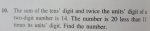# sum of the tens digit and twice the units digit of a two digit number is 14.

#### allegansveritatem

##### Full Member
I have a simple problem here that I can't seem to get to come out right. Should be easy...but I keep getting fractional results where they have no business showing up. The problem is this: the sum of the tens digit and twice the units digit of a two digit number is 14. The number is 20 less than 11 times the units digit. What is the number.
I set this up thus:

t + 2u=14
10t+ u= 11u-20

which reduced to:

t+2u=14
10t-10u=-20

I multiplied the top equation by 5

5t + 10u=70
10t - 10u=-20

This works out to:

15t=50 or t=50/15

How can this be right? I have gone over this about 5 times and can't get around this result. Can anyone see where I am straying from the primrose path of truth?

#### Dr.Peterson

##### Elite Member
I have a simple problem here that I can't seem to get to come out right. Should be easy...but I keep getting fractional results where they have no business showing up. The problem is this: the sum of the tens digit and twice the units digit of a two digit number is 14. The number is 20 less than 11 times the units digit. What is the number.
I set this up thus:

t + 2u=14
10t+ u= 11u-20

which reduced to:

t+2u=14
10t-10u=-20

I multiplied the top equation by 5

5t + 10u=70
10t - 10u=-20

This works out to:

15t=50 or t=50/15

How can this be right? I have gone over this about 5 times and can't get around this result. Can anyone see where I am straying from the primrose path of truth?
I get the same result by a different method.

Either the problem itself is wrong, or you copied something wrong. Please check!

If it said, "the sum of the units digit and twice the tens digit, it would work.

#### allegansveritatem

##### Full Member
I get the same result by a different method.

Either the problem itself is wrong, or you copied something wrong. Please check!

If it said, "the sum of the units digit and twice the tens digit, it would work.
I don't have my book with me right now but I will check it tomorrow to make sure. I think I got it right...but I know myself too well to be certain. Thanks for working it out.

#### allegansveritatem

##### Full Member
I get the same result by a different method.

Either the problem itself is wrong, or you copied something wrong. Please check!

If it said, "the sum of the units digit and twice the tens digit, it would work.
Here is a photo of the problem. I had it right. I guess Dr Blixer delegated one of his grad students to draw up the problems for this section. I think that must be the case generally because I detect a lot of irony and humor in some of these word problems that seem more like the irony and humor of a bored student than that of a bored professor.